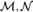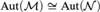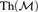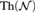Home

# AUTOMORPHISM GROUPS OF COUNTABLE ARITHMETICALLY SATURATED MODELS OF PEANO ARITHMETIC

## Abstract

If${\cal M},{\cal N}$ are countable, arithmetically saturated models of Peano Arithmetic and${\rm{Aut}}\left( {\cal M} \right) \cong {\rm{Aut}}\left( {\cal N} \right)$ , then the Turing-jumps of${\rm{Th}}\left( {\cal M} \right)$ and${\rm{Th}}\left( {\cal N} \right)$ are recursively equivalent.

Hide All

# AUTOMORPHISM GROUPS OF COUNTABLE ARITHMETICALLY SATURATED MODELS OF PEANO ARITHMETIC

## Metrics

### Full text viewsFull text views reflects the number of PDF downloads, PDFs sent to Google Drive, Dropbox and Kindle and HTML full text views.

Total number of HTML views: 0
Total number of PDF views: 0 *Loading metrics...

### Abstract viewsAbstract views reflect the number of visits to the article landing page.

Total abstract views: 0 *Loading metrics...

* Views captured on Cambridge Core between <date>. This data will be updated every 24 hours.

Usage data cannot currently be displayed